Test: Basic Trigonometric Formula

# Test: Basic Trigonometric Formula - Commerce

Test Description

## 15 Questions MCQ Test Mathematics (Maths) Class 11 - Test: Basic Trigonometric Formula

Test: Basic Trigonometric Formula for Commerce 2023 is part of Mathematics (Maths) Class 11 preparation. The Test: Basic Trigonometric Formula questions and answers have been prepared according to the Commerce exam syllabus.The Test: Basic Trigonometric Formula MCQs are made for Commerce 2023 Exam. Find important definitions, questions, notes, meanings, examples, exercises, MCQs and online tests for Test: Basic Trigonometric Formula below.
Solutions of Test: Basic Trigonometric Formula questions in English are available as part of our Mathematics (Maths) Class 11 for Commerce & Test: Basic Trigonometric Formula solutions in Hindi for Mathematics (Maths) Class 11 course. Download more important topics, notes, lectures and mock test series for Commerce Exam by signing up for free. Attempt Test: Basic Trigonometric Formula | 15 questions in 15 minutes | Mock test for Commerce preparation | Free important questions MCQ to study Mathematics (Maths) Class 11 for Commerce Exam | Download free PDF with solutions
 1 Crore+ students have signed up on EduRev. Have you?
Test: Basic Trigonometric Formula - Question 1

### cos 68° cos 8° + sin 68° sin 8° = ?

Detailed Solution for Test: Basic Trigonometric Formula - Question 1

We know,
cosA cosB + sinA sinB = cos(A-B)

cos 68° cos 8° + sin 68° sin 8° = Cos (68-8) = Cos60°
=1/2

Test: Basic Trigonometric Formula - Question 2

### In a triangle ABC, tan A/2 = 5/6, tan B/2 = 20/37, then tan C/2 is equal to:

Detailed Solution for Test: Basic Trigonometric Formula - Question 2

In triangle ABC,
► Sum of all three angles = 1800
► A + B + C = 180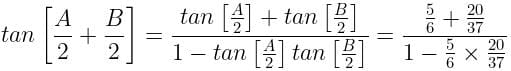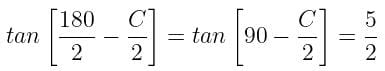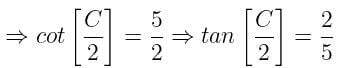Test: Basic Trigonometric Formula - Question 3

### In a triangle ABC, cosec A (sin B cos C + cos B sin C) equals:

Detailed Solution for Test: Basic Trigonometric Formula - Question 3

cosec A (sin B cos C + cos B sin C) = cosec A × sin(B+C)
= cosec A × sin(180 – A)
= cosec A × sin A
= cosec A × 1/cosec A
= 1

Test: Basic Trigonometric Formula - Question 4

The value of Cos75°is equal to

Detailed Solution for Test: Basic Trigonometric Formula - Question 4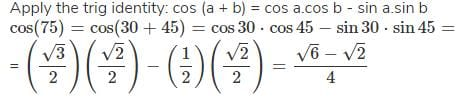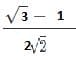Test: Basic Trigonometric Formula - Question 5

Sin A = 1/√10 , Sin B = 1/√5 If A and B are both acute angles, then, A+B =?

Detailed Solution for Test: Basic Trigonometric Formula - Question 5

We know that:
Sin θ = Opposite / Hypotenuse

∴ SinA = 1/√10
CosA= 3/√10
similarly, SinB = 1/√5
CosB= 2/√5

Multiply:
Cos(A+B)= CosA x CosB - SinA x SinB

Substituting the value in above equation we get:

= 3/√10 x 2/√5 - 1/√10 x 1/√5
= 6/√50 - 1/√50
= 6-1/5√2. ........(√50=5√2)
= 1/ √2

we know that, sin 45 = 1/ √ 2 therefore
sinθ / cosθ = 45

Test: Basic Trigonometric Formula - Question 6

If 3 × tan(x – 15) = tan(x + 15), then the value of x is:

Detailed Solution for Test: Basic Trigonometric Formula - Question 6

3 × tan (x – 15) = tan (x + 15)
⇒ tan(x + 15) / tan(x – 15) = 3/1

► {tan (x + 15) + tan (x – 15)} / {tan (x + 15) – tan (x – 15)} = (3 + 1) / (3 – 1)

► {tan (x + 15) + tan (x – 15)} / {tan (x + 15) – tan (x – 15)} = 2

sin(x + 15 + x – 15) / sin(x + 15 – x + 15) = 2
sin 2x / sin 30 = 2
sin 2x / (1/2) = 2
2 × sin 2x = 2
Sin 2x = 1

∴ Sin 2x = Sin 90
2x = 90
x = 45

Test: Basic Trigonometric Formula - Question 7

The value of tan 20 × tan 40 × tan 80 is

Detailed Solution for Test: Basic Trigonometric Formula - Question 7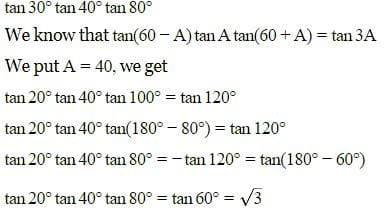Test: Basic Trigonometric Formula - Question 8

Chose which of the following expressions equals sinA + cosA.

Detailed Solution for Test: Basic Trigonometric Formula - Question 8

sinA + cosA = √2(sinA/√2+cosA/√2)
=√2(sinAcos(π/4)+cosAsin(π/4))
=√2sin(A+π/4)
by using sin(A+B) formula

Test: Basic Trigonometric Formula - Question 9

If acos x + bsin x = c, then the value of (asin x – bcos x)²  is:

Detailed Solution for Test: Basic Trigonometric Formula - Question 9

(acos x + bsin x)² + (asin x – bcos x)² = a² + b²
⇒ c² + (asin x – bcos x)² = a² + b²
⇒ (asin x – bcos x)² = a² + b² – c²

Test: Basic Trigonometric Formula - Question 10

sin(60° + A) cos(30° – B) + cos(60° + A) sin(30° – B) is equal to:

Detailed Solution for Test: Basic Trigonometric Formula - Question 10

L.H.S. = sin(60+A)cos(30−B)+cos(60+A)sin(30−B)
= sin[(60+A)+(30−B)]            (Using, sin(A+B)sinAcosB+cosAsinB)
= sin(90+A−B)
= sin(90+(A−B))
= cos(A−B)            (Using, sin(90+θ)=cosθ)       = R.H.S.Hence Proved.

Test: Basic Trigonometric Formula - Question 11

If cos a + 2cos b + cos c = 2 then a, b, c are in

Detailed Solution for Test: Basic Trigonometric Formula - Question 11

cos A + 2 cos B + cos C = 2
⇒ cos A + cos C = 2(1 – cos B)

2 cos((A + C)/2) × cos((A-C)/2 = 4 sin²(B/2)

2 sin(B/2) cos((A-C)/2) = 4 sin² (B/2)
cos((A-C)/2) = 2 sin (B/2)
cos((A-C)/2) = 2 cos((A+C)/2)
cos((A-C)/2) – cos((A+C)/2) = cos((A+C)/2)

2 sin(A/2) sin(C/2) = sin(B/2)
⇒ 2 {√(s-b)(s-c)√bc} × {√(s-a)(s-b)√ab} = √(s-a)(s-c)√ac

2(s – b) = b
a + b + c – 2b = b
a + c – b = b
a + c = 2b

Test: Basic Trigonometric Formula - Question 12

sin(n+1)A sin(n+2)A + cos(n+1)A cos(n+2)A =

Detailed Solution for Test: Basic Trigonometric Formula - Question 12

sin(n+1)Asin(n+2)A + cos(n+1)Acos(n+2)A = cos (n+1)Acos(n+2)A + sin(n+1)Asin(n+2)A = cos{A(n+2-n-1)} = cos (A.1) = cos A

Test: Basic Trigonometric Formula - Question 13

cos(π/4 - x) cos (π/4 - y) -sin(π/4 - x) sin(π/4 - y) =

Detailed Solution for Test: Basic Trigonometric Formula - Question 13

Cos(π/4-x)cos (π/4-y) - sin (π/4-x) sin(π/4-y)

= CosA*Cos B - Sin A*Sin B
= Cos (A+B)
= cos(π/4-x+π/4-y)
= cos(π/2-x-y)
= cos{π/2 - (x+y)}
= sin(x+y)

Test: Basic Trigonometric Formula - Question 14

The value of tan 3A – tan 2A – tan A is

Detailed Solution for Test: Basic Trigonometric Formula - Question 14

3A= A+ 2A
⇒ tan 3A = tan (A + 2A)
⇒ tan 3A = (tan A + tan 2A) / (1 – tan A . tan 2A)
⇒ tan A + tan 2A = tan 3A – tan 3A x tan 2A . tan A
⇒ tan 3 A – tan 2A – tan A = tan 3A . tan 2A . tan A

Test: Basic Trigonometric Formula - Question 15

Which of the following is incorrect:

## Mathematics (Maths) Class 11

157 videos|210 docs|132 tests
Information about Test: Basic Trigonometric Formula Page
In this test you can find the Exam questions for Test: Basic Trigonometric Formula solved & explained in the simplest way possible. Besides giving Questions and answers for Test: Basic Trigonometric Formula, EduRev gives you an ample number of Online tests for practice

## Mathematics (Maths) Class 11

157 videos|210 docs|132 tests(Scan QR code)